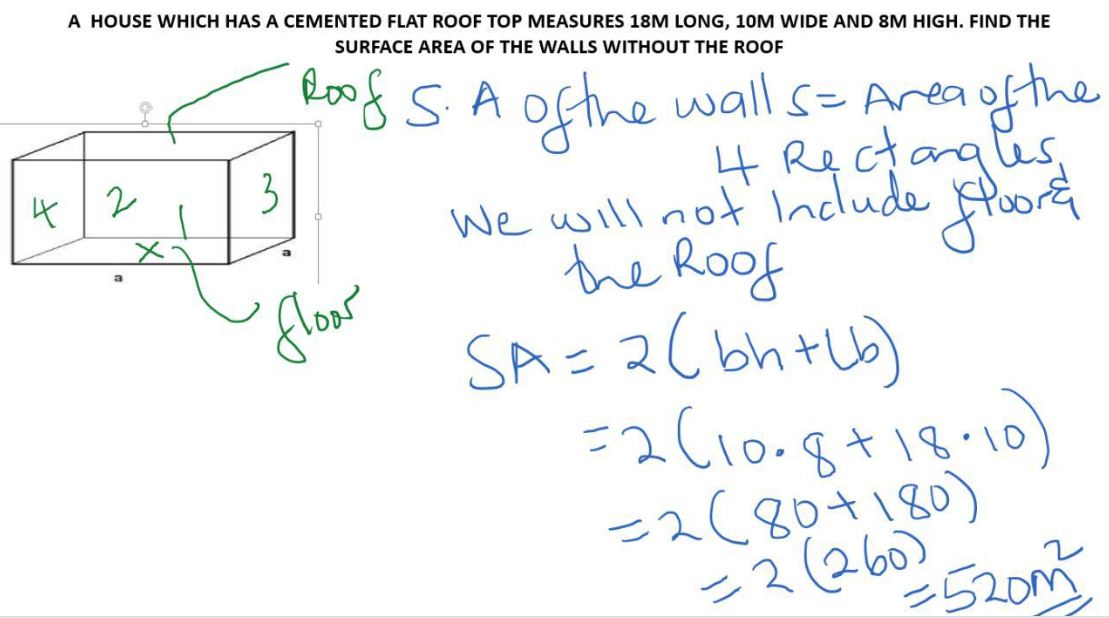Surface Area of a Cuboid

A cuboid is a solid with 6 rectangular faces.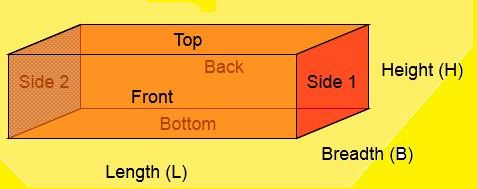A cuboid is made up of 6 rectangles.

The area of a rectangle is obtained by = L×W

Surface area of a cuboid is given by adding up the areas of the six rectangles.

= L × W + L × W + L × W + L × W + L × W + L × W

SA of a Cuboid is given by: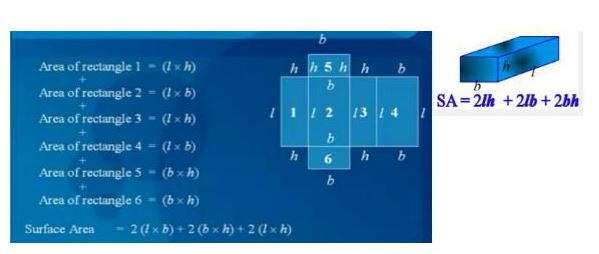#### Activity 1

Attempt to answer the question given here before moving on.

A cuboid measures 30 cm by 10 cm by 8 cm. Calculate it's total surface area.

#### Solution 1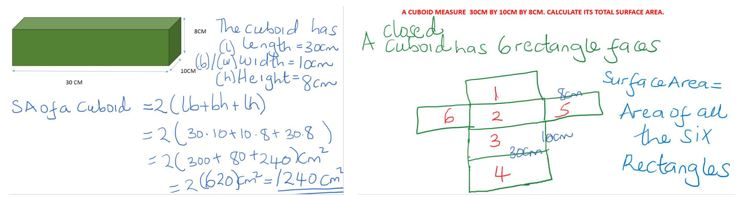#### Activity 2

Attempt to solve the problem 2 before moving on

The length of an open cuboid is 65 mm. it's width is 4 cm and it's height is 6 cm. Find it's total surface area in cm.

#### Solution 2

Study the solution below and compare with your own work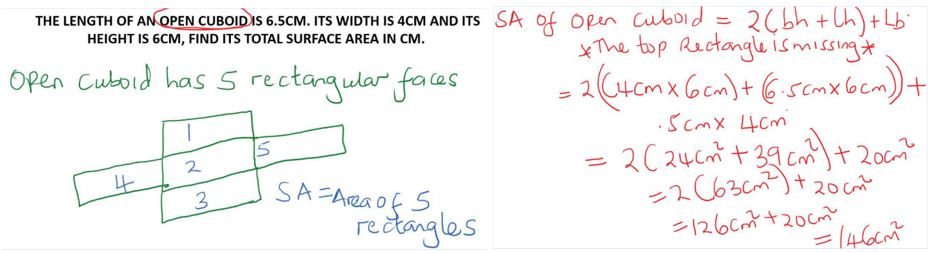#### Activity 3

Attempt to answer the question below before moving on.

A house which has a cement flat roof top measures 18m long, 10mm wide and 8m high. Find the surface area of the walls without the roof.

#### Solution 3

Study the solution below and compare your own work to the solution provided